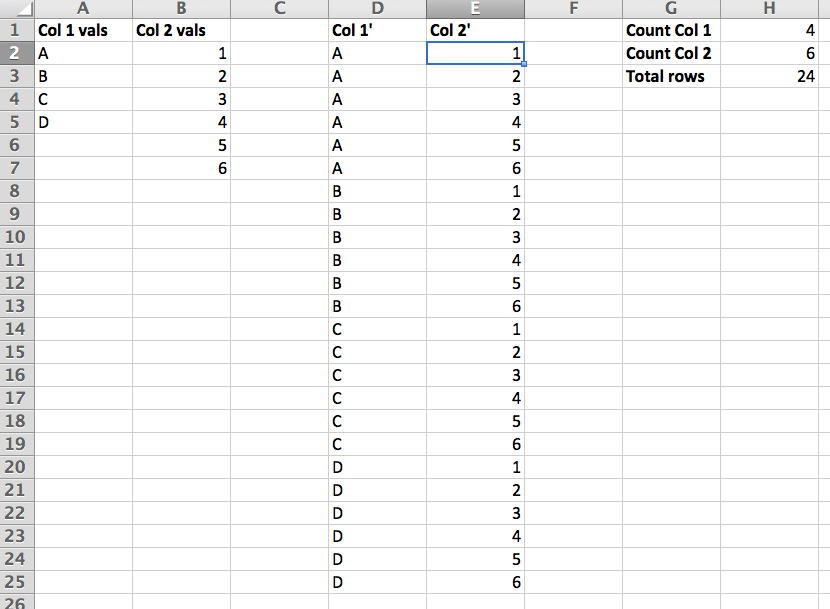# Gap fills and cross joins in Excel

2014-05-03 2 min read

During my consulting years I’ve done a ton of Excel and noticed people getting frustrated by two seemingly simple operations. The first is getting a worksheet with gaps in a column and needing to fill it with values from the cells above and the second is doing a cross join between two sets of values.

The solution to the gap filling can be done by explaining the solution in such a way that it can be implemented via an Excel formula. The best I could come up with is “If a gap is a value, take the value of the closest non empty cell above it, otherwise keep its value.” We can create a formula in another column that takes this approach and after coming up with the new cell values and pasting them over the originals. In the image below, the formula in cell D2 is =A1 and the formula in D3 is =IF(A3="",D2,A3) with D4 down being relative copies of D3.The cross join problem is similar - we have two sets of values and need to enumerate each combination. The key point is realizing that we know what the values should be in a particular row and deriving the formula to get those values. My approach uses integer division to get the value in the first column and modulo to get the value in the second column although any function that’s deterministic should work. In the image below, the formula in cells D2 through D25 is =INDEX($A$2:$A$5,(ROW()-2)/$H$2+1) and the formula in cells E2 through E25 is =INDEX($B$2:$B$7,MOD(ROW()-2,$H$2)+1).The file with the two approaches can be grabbed here.